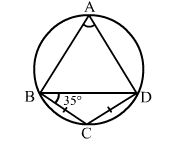# In the give figure, ABCD is a cyclic quadrilateral in which BC = CD and ∠CBD = 35°.

Question:

In the give figure, ABCD is a cyclic quadrilateral in which BC = CD and CBD = 35°. Then, ∠BAD = ?
(a) 65°

(b) 70°
(c) 110°
(d) 90°Solution:

(b) 70°
BC = CD (given)
⇒ BDC = ∠CBD = 35°
In
Δ BCD, we have:
∠BCD +  BDC + ∠CBD = 180°     (Angle sum property of a triangle)
⇒ ∠BCD + 35° + 35° = 180°
⇒ ∠BCD = (180° - 70°) = 110°
In cyclic quadrilateral ABCD, we have: